Inventory Management Problem

1. Introduction

Inventory management is a key problem in several industries, (car renting, storehouse space renting, etc.). It consists of managing a given fleet of equipment in order to satisfy requests to use it. When requests exceed the stock of available equipment, a decision has to be made, either to subcontract some requests to another provider or to purchase new pieces of equipment. The main difficulty lies in the fact that a subcontracted request must be subcontracted for all the duration of the request. For example, if a subcontracted car is rented to a given customer, this customer will keep the subcontracted car for all the duration of the rental. In the following, we propose a set of benchmark problem instances, derived from real-world inventory management problems.

2. Technical description of the problem

2.1. Informal description

Let us consider a warehouse that manages a stock of different types of equipment. The warehouse receives requests from customers asking for some resources of some type for a given period. Basically a request is characterized by its place in time (start and end time), by the required resource type, and by the number of resources. We suppose that all requests are known for a given period (for example, a few months or a year).

The satisfaction of all requests is mandatory. If there are not enough resources available in stock, the manager has to decide either to buy new resources or to subcontract some requests to another provider. Given the cost of all operations (rent, purchase, allocation from available stock), decisions have to made in such a way that the sum of costs is minimized.

For some resource types, items can be replaced with items of other resource types (this relation is transitive). For example, a customer requiring a small car may be allocated a medium or a large car. Obviously these replacements have a cost while customers have the same bill with or without replacement. However, in general, subcontracting a resource (or another resource) can be more expensive than replacing it with a more expensive one that is available in stock.

In general, after a given time of actual use, each resource has to be maintained. We can do the maintenance before, but after this time of use, the maintenance is mandatory. The duration of the maintenance and the number of maintenance units (for example, workers) required for the maintenance of a given resource type are known. Thus, in order to allocate resources from existing stock, some constraints have to be satisfied. On the one hand, no resource can be used more than its maximal using time without maintenance; on the other hand, equipment maintenance is planned so that the capacity of the maintenance workshop is never exceeded. Let us note that, for some resource types, maintenance is not an issue.

The main problem consists in determining

• which requests have to be subcontracted,
• which resources are allocated to those requests that are not subcontracted,
• when to buy new items, and
• at what time the resources are maintained,

so that all of the constraints are satisfied and the entire cost of the operations is minimal.

The main source of complexity of this problem lies in the fact that, for each request, the same pieces of equipment (whether subcontracted or taken from the stock) will be used throughout the duration of the request. For example, if customer X requests a resource from May 7 to May 17, customer Y requests a resource from May 13 to May 26, and only one resource is available in stock, one must decide either to subcontract a resource for X from May 7 to May 17, or to subcontract a resource for Y from May 13 to May 26. It is not possible to merely subcontract a resource for Y from May 13 to May 17, and replace the subcontracted resource by the resource originating from the stock when it returns from X, because the cost of such a replacement would be too high. (For example, a rental car company cannot replace the car used by one of its customers (Y) in the course of the rental (on May 17). This would be too inconvenient for the customer.) As a result, the problem does not merely consist of identifying the time periods over which the demand (sum of the requests) exceeds the supply available in stock. One must also select which requests to subcontract, so as to minimize the total cost associated with the requests under consideration. In the X versus Y example above, it would be wiser to subcontract X’s request, since the subcontract would run only for 10 days (instead of 13 if Y’s request was subcontracted). However, should another customer Z place a request for a resource from May 20 to May 29, it would become wiser to subcontract Y’s request, rather than both X’s request and Z’s request. This shows that the best global decision does not derive from the best local decisions: when only X and Y are considered, the best solution consists of subcontracting X’s request; when only Y and Z are considered, the best solution consists of subcontracting Z’s request; yet, when X, Y, and Z are considered, the best solution consists of subcontracting Y’s request, rather than X’s and Z’s.

The problem is further complicated by the other types of decisions that could or should be made: purchasing new resources, providing a bigger or more sophisticated resource (e.g., a bigger car) in response to a given request, and scheduling the maintenance of each individual resource. When maintenance constraints apply, they appear to be highly critical as (1) a piece of equipment cannot be allocated to a request if it needs to be maintained, (2) the maintenance facility has limited capacity, which means that a piece of equipment may have to wait (queue) before being maintained. These two factors impair the quantity of equipment that can actually be allocated from the stock. Hence, different maintenance schedules may lead to more or less subcontracting. In practice, this means that equipment allocation and maintenance scheduling decisions must be tightly coordinated, which in turn makes it necessary to decide exactly which individual pieces of equipment are allocated to each request. The following formal problem description distinguishes each individual piece of equipment. However, units of the same resource type can be considered equivalent when maintenance is not an issue.

2.2. Formal description

As we mentioned in the previous section, a time period is considered for each problem. At the beginning of this period, the equipment in stock may have been used without maintenance and some requests are being handled. For the sake of simplicity we will not consider this initial state which has no effect on the complexity of the problem.

In the following, we give a formal description of the inventory management problem.

Let R = {r1, r2, ..., rk} be a set of resource types. We say that the resource type ri subsumes the resource type rj (ri p rj) if any unit of type rj can be substituted by any unit of type ri. The Inventory Management Problem is defined by the following data:

• [Start, End] - discrete time interval such that Start, End Î N and Start £ End
• (R, p ) - set of resource types and the subsumption relation defined on it

for all r in R,

Stock(r,Start-1) - set of items of type r available in stock at time (Start - 1)

Utime(r) - maximal time of use of an item without maintenance

Mtime(r) - duration of the maintenance of an item

Mcap(r) - number of capacity units required for the maintenance of a

resource of type r.

Maxbuy(r) - maximal number of items that can be bought during the

period

Crent(r) - external renting price (per time unit)

Cafix(r) - fixed cost of the allocation of an item to an order

Catd(r) - time dependent cost of the allocation of an item to an order

(per time unit)

Cbuy(r) - period length dependent purchase cost of an item

Cstock(r) - fixed cost of an item (used or not) in stock (per time unit)

Cmaint(r) - cost of maintenance of an item

• MCAP - capacity of the maintenance workshop
• O - set of orders

for all o in O,

st(o) - start time of o, such that st(o) Î N and Start £ st(o) < End

et(o) - end time of o, such that et(o) Î N , st(o) < et(o) £ End

rtype(o) - required resource type

rq(o) - required number of rtype(o) for the order o

A solution is a valuation for the functions

• rent(r,o): R ´ O ® N - number of items of type r from external

rentals (subcontracts) for the order o

• buy(r,t): R ´ [Start,End] ® P (r) - set of items of type r bought at time t

• maint(r,u): R ´ Stock(r,End) ® P (N ) - set of starting time of maintenance for the

item u of type r

• alloc(r,o): R ´ O ® P (r) - set of items of type r allocated to the order

o (alloc(r,o) Í Stock(r,st(o))

such that the following constraints are verified:

1. allocated and rent resources correspond to the required resource type of the order:
2.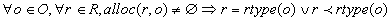and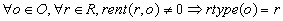3. all orders are satisfied:
4.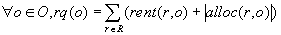6. r) items of type r are purchased during the period:7. the same item is not allocated to two orders which intersect in time. For all o and o’ different intersecting orders in O (that is o ¹ o’ and st(o) £ st(o’) < et(o)):
8.9. for all items u of type r there is a maintenance after at most Utime(r) effective use. Let ord denote the ordered inverse of the function alloc: ord(u) is an ordered set of orders o such that u belongs to alloc(r,o) for some r in R. ord(u) is ordered by the starting time (st) of its elements. Let S(u) denote the set of consecutive subsets (intervals) of ord(u), and for all s in S(u), l(s) denote the sum of the duration of the orders in S(u), st(s) the starting time of the first order in s and et(s) the end time of the last order in s.
10.However, if an order o requests a resource of type r and if the duration of o is greater than Utime(r), a resource u of type r can be assigned to o provided that o is the only order scheduled between two consecutive maintenances of u including o. In other terms, the above constraint does not apply when s = {o}.

11. at each moment, the sum of the maintenance capacities required by the items in maintenance should not exceed the capacity of the maintenance workshop.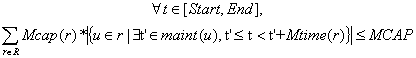An optimal solution is a solution where the cost function F is minimal. The function F is composed of three parts. First, the cost of external rentals and resource allocations from existing stock:,

where d(o) = et(o) - st(o), then the cost of purchase:,

and the cost of the maintenance: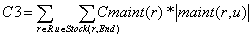The cost function is defined as the sum of the three previous functions: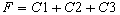The overall problem is NP-hard in the strong sense. Indeed, given an instance of the multiprocessor scheduling problem  i.e., a set of tasks T, a capacity MCAP, a duration d(t) for each task, and an overall deadline D, one can easily create an instance of the inventory management problem which accepts a solution with no external rental if and only if the multiprocessor scheduling problem accepts a solution (for each task t in T, create a resource type rt with Mtime(rt) = d(t), Utime(rt) = 1, Mcap(rt) = 1, and two orders for this resource type, the first finishing at time 0, and the second starting at time D).

3. Data

3.1. Input and output data format

A series of instances of the above problem is available on the web site http://www-icparc.doc.ic.ac.uk/chic2/benchmarks/inventory.htm. This section describes the input and output data format for these benchmarks.

3.1.1. Input data

The input file is composed of four parts in the following order:

• definition of the global variables of the problem (period and capacity of the maintenance workshop)

globals(start,end,mcap)

where start, end and mcap are integer. A negative value of mcap means that maintenance is not considered.

• definition of resource types as a sequence of individual resource type definition. A resource type is defined in the following way:

resource(rtype_id, Stock, Utime, Mtime, Mcap, Crent, Cafix, Catd,

where rtype_id is an integer identifier of the resource type and Stock, Utime, ... are integers correspondig to the previously defined variables Stock, Utime, .... For example:

resource(1, 2, 150, 10, 2, 15, 13, 5, 1, 1, 4, 0)

is a resource type definition where the resource type identifier (rtype_id) is 1, the available stock (Stock) of this resource type is 2, etc. If there is no maintenance (mcap is negative in globals(...)) the parameters Utime, Mtime, Mcap and Cmaint are ignored. If the last parameter (Maxbuy) is negative, the number of purchased items is not limited.

• definition of the substituability relation by a sequence of blocks

substituable(rtype_id1, rtype_id2)

where rtype_id1 and rtype_id2 are two existing resource type identifiers. It means that all units of the resource type identified by rtype_id1 can be substituted by units of the type identified by rtype_id2. For example:

substituable(2, 1)

means that each resource of type 2 can be substituted by each resource of type 1.

If the substituability relation is empty then this part can be ignored.

• definition of the set of orders by a sequence of individual order definition:

order(order_id, st, et, rq, rtype_id)

where order_id, st, et, rq, rtype_id are integers and correspond to the order identifier, start time, end time, required quantity and required resource type of the order. For example:

order(2, 0, 35, 2, 1)

means that the order 2 starts at time 0, ends at time 35, and requires 2 resources of type 1.

3.1.2. Output data

If there is maintenance, we consider that a unique integer identifier is attributed to each unit of each resource type. Since at the beginning the units are equivalents, the identifiers have to be defined by the programmer and they appear only in the output file. If maintenance is not considered, the output file is defined by three blocks:

• rentals (predicate rent), a sequence of lines of format

rent(rtype_id, order_id, rnumber)

where rnumber is the number of items of type rtype_id rent for the order identified by order_id.

• purchase (predicate buy), a sequence of lines of format

where bnumber is the number of items of type rtype_id bought at time t.

• allocations (predicate alloc), a sequence of lines of format:

alloc(rtype_id, order_id, anumber)

which shows the number of items of type rtype_id which are allocated from existing stock to the order identified by order_id.

If maintenance is considered, the output file is defined by four blocks:

• rentals (predicate rent) - as in the previous case
• purchase (predicate buy), a sequence of lines of format

which shows that an item of type rtype_id identified by item_id was bought at time t.

• allocations (predicate alloc), a sequence of lines of format:

alloc(rtype_id, order_id, item_id)

which shows that an item of type rtype_id identified by item_id was allocated to the order identified by order_id.

• maintenance (predicate maint), a sequence of lines of format:

maint(rtype_id, item_id, t)

where t is the starting time of a maintenance of the item item_id of type rtype_id.

3.2. A small example

Let us consider the following input data:

globals(0,100,-1)

resource(1,4,-1,-1,-1,20,20,4,1000,0,-1,1)

order(1,0,35,2,1)

order(2,5,30,3,1)

order(3,32,87,5,1)

In this example, 4 resources of type 1 are available in stock. Maintenance is not considered. In the time interval [0,100] we have to satisfy three orders. These orders are defined by their starting time (st), end time (et), required resource type (rtype), and required quantity (rq):

st(1) = 0, et(1) = 35, rtype(1) = 1, rq(1) = 2,

st (2) = 5, et (2) = 30, rtype (2) = 1, rq(2) = 3,

st (3) = 32, et (3) = 87, rtype (3) = 1, rq(3) = 5It is clear that we cannot satisfy all of these orders from existing stock, so we have to rent some resources. In this example, Crent = 20, Cafix = 20, and Catd = 4. Thus, for example if we rent two resources for the order 1 the cost is 2*(35-0)*20 = 1400 while if we allocate two resources from our stock the cost is 2*(Cafix + (35-0)*Catd)= 320.A resource (from existing stock or from external rent) has to be assigned to an order o from st(o) to et(o). Meanwhile we cannot replace resources (replacement is considered very expensive). Thus, we cannot just rent one resource for the period [5,30], three resources for the period [32,35] and one resource for the period [35,87], which would be ideal.

A possible solution of our example is (in output text format):

rent(1,2,1)

rent(1,3,3)

alloc(1,1,2)

alloc(1,2,2)

alloc(1,3,2)The cost of this assignment is 320 + 740 + 3780 = 4840. However we can find a better solution (which is optimal) of cost 1400 + 360 + 2060 = 3820:

rent(1,1,2)

rent(1,3,1)

alloc(1,2,3)

alloc(1,3,4)4. Benchmark problems

We propose eight instances (files ".tes") of the inventory management problem with two variants for each (*b.tes and *nb.tes). For each problem instance, we consider two variants:

• purchase is authorized (the parameter Maxbuy is greater than 0).
• purchase is not authorized (the parameter Maxbuy is equal to 0).

For example, the instances I808b.tes and I808nb.tes are different only in the value of the parameter Maxbuy. For each instance, we give a possible solution (not necessarily the optimal). The solution files have the same name as the problem files with the extension ".rsl". The following table resumes the cost of the given solutions.

Instances with purchase

 Instances I808b I1507b I2007b I2007bisb I2109b I2109bisb I20012b I16012b cost 1223782 2898008 6121844 4205250 6564389 4614876 5405253 3584508

Instances without purchase

 Instances I808nb I1507nb I2007nb I2007bisnb I2109nb I2109bisnb I20012nb I16012nb cost 1288400 3411081 5922665 4707575 7039182 5317185 6157716 3970174